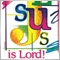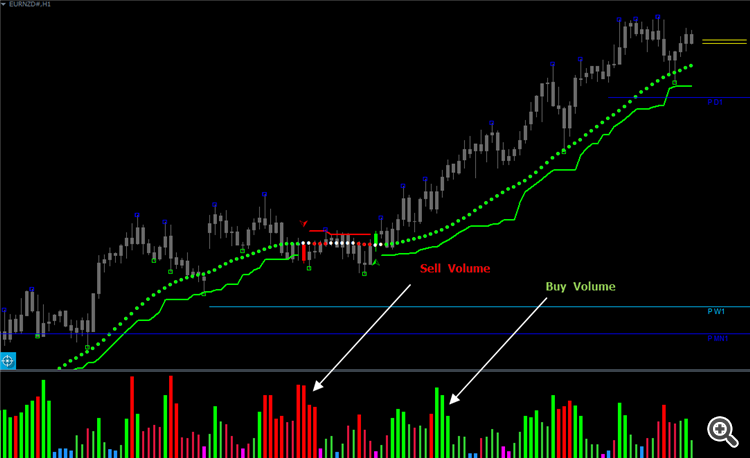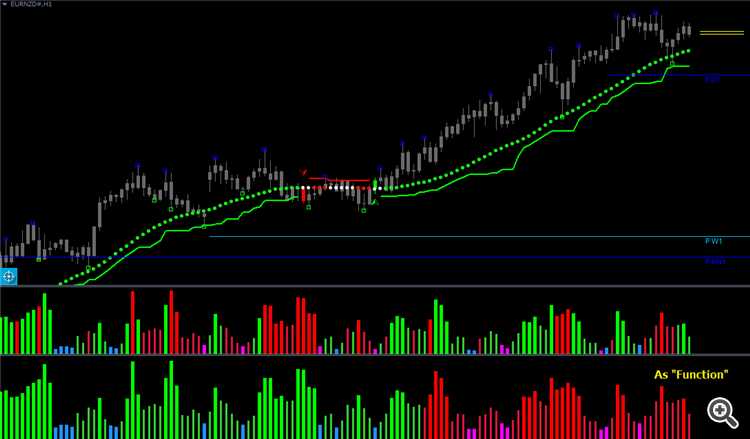# Indicator logic as function returns wrong data103

Hi,

I coded a volume indicator that helps me "categorize" tick volume as buy and sell volume,In an effort to streamline my coding workflow and avoid calling the indicator above using iCustom, which is resource heavy when viewing multiple charts and multiple timeframes, I decided to transfer the logic into a single function which resides in an .mqh header file. The result is simply bogus;While I cant share the full source code because its proprietary, I can share code snippets to show the steps I take.

Logic;

```//BUFFERS

double         upc[];// cumulative up volume
double         dnc[];//cumulative up volume
double         vo[];// volume

int OnInit()
{

//empty buffer is a custom function to speed up declaration of buffers
emptybuffer(0, upc);
emptybuffer(1, dnc);
emptybuffer(3, vo);
}

//+------------------------------------------------------------------+
//|                                                                  |
//+------------------------------------------------------------------+
int OnCalculate(const int rates_total,
const int prev_calculated,
const datetime & time[],
const double & open[],
const double & high[],
const double & low[],
const double & close[],
const long & tick_volume[],
const long & volume[],
{
int trend = 0;
int limit = MathMax(rates_total - 50 - prev_calculated, 50);
int i = 0;
datetime lbar = time;

if(lbar != time && !IsStopped()) // prevents the indicator from repainting
{
lbar = time;

//first loop to fill the volume buffer
for(i =  limit; i >= 0 && !IsStopped(); i--)
{
vo[i] = EMPTY_VALUE;
vo[i] = NormalizedVolume(i);

}

//second loop to "categorize" the volume as buy and sell
for( i =  limit; i >= 0 && !IsStopped(); i--)
{

/*

"categorization" algorithm goes here.
Returns the value of "trend" variable.

*/
if(trend == 1)
{
upc[i] = vo[i] ;
dnc[i] = 0;

}

else if(trend == -1)
{
dnc[i] = vo[i];
upc[i] = 0;

}
else
{
dnc[i] = 0;
upc[i] = 0;

}
//smoothing
double diffBufferx = iMAOnArray(upc, 0, 5, 0, MODE_LWMA, i) - iMAOnArray(dnc, 0, 5, 0, MODE_LWMA, i);

double diffa = 0;

if(diffBufferx == diffa)
//Draw neutral volume

else if(diffBufferx < diffa)
//Draw bearish volume

else if(diffBufferx > diffa)
//Draw bullish volume

else
//Draw neutral volume

}

}```

As a function;

```// Contents of filter-cores.mqh

//+------------------------------------------------------------------+
//|  initialize buffers                                              |
//+------------------------------------------------------------------+

//this is called in OnInit()

void InitCoreBuffers(bool as_sries = true)
{

if(as_sries)
{

ArraySetAsSeries(fnv, true); //volume buffer
ArraySetAsSeries(upvc, true); // up volume
ArraySetAsSeries(dnvc, true); // down volume
}

ArrayResize(fnv, Bars);
ArrayResize(upvc, Bars);
ArrayResize(dnvc, Bars);

ArrayInitialize(fnv, EMPTY_VALUE);
ArrayInitialize(upvc, EMPTY_VALUE);
ArrayInitialize(dnvc, EMPTY_VALUE);

}

//+------------------------------------------------------------------+
//|  Categorize volume                                               |
//+------------------------------------------------------------------+

//This is called in OnCalculate()

int CoreVolumeFilter(double &vbuffer[],double &vup[], double &vdn[], int i, ENUM_TIMEFRAMES timeframe = PERIOD_CURRENT, string symbol = NULL, int vperiod = 50, int smoothing = 5)
{

vup[i] = EMPTY_VALUE;
vdn[i] = EMPTY_VALUE;

int retVtype = 0, trend = 0;
/*

Categorization algorithm goes here.
Returns the value of "trend" variable.

*/
if(trend == 1)
{
vup[i] = vbuffer[i];
vdn[i] = 0;

}

else if(trend == -1)
{
vup[i] = vbuffer[i];
vdn[i] = 0;

}
else
{
vup[i] = 0;
vdn[i] = 0;

}
//smoothing
diffBuffer = iMAOnArray(vup, 0, smoothing, 0, MODE_LWMA, i) - iMAOnArray(vdn, 0, smoothing, 0, MODE_LWMA, i);

double diffa = 0;//

if(diffBuffer == diffa)
{
retVtype =0;
//returns neutral volume type
}
else if(diffBuffer < diffa)
{
retVtype =-1;
//returns bearish volume type
}
else if(diffBuffer > diffa)
{
retVtype =1;
//returns bullish volume type

}
else
{
retVtype =0;
//returns neutral volume type

}

return  retVtype;
}```

Calling the function above in OnCalculate();

```/*
The volume buffer
fnv[] is filled by a separate function in a separate loop just like the original code,
The volume returned is accurate, what fails is the categorization and I cant seem to find the problem.

*/
int volume_type = CoreVolumeFilter(fnv,upvc,dnvc,i);

//draw  bullish /bearish buffers
```

Any help will be highly appreciated.103

If you can't show all the source code, trace it yourself.

We don't know even if we see only a part of it.

It is very important to learn how to debug.

Just for clarity, I simply want to know if I took the correct steps to "functionalize"(for lack of a better word) the indicator, I'm more interested in the "framework" to do such a thing properly. This is the second indicator I've attempted to "functionalize" and the results are the same, just bogus. What mql coding ethic/structure am I not following ?

Thanks for the link on debugging, I'll familiarize myself with it over the weekend, however,  is it just me or is metatraders' debugging framework clumsy ?

Thank you Unanda.